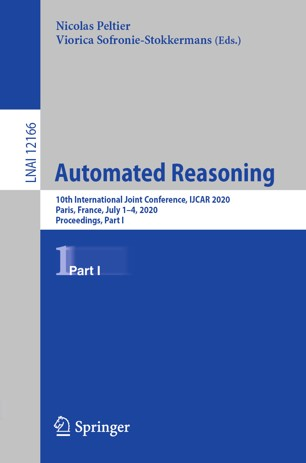• A
• A
• A
• ABC
• ABC
• ABC
• А
• А
• А
• А
• А
Regular version of the site

## Soft subexponentials and multiplexing

P. 500-517.
Kanovich M., Kuznetsov S., Nigam V., Scedrov A.

Linear logic and its refinements have been used as a specification language for a number of deductive systems. This has been accomplished by carefully studying the structural restrictions of linear logic modalities. Examples of such refinements are subexponentials, light linear logic, and soft linear logic. We bring together these refinements of linear logic in a non-commutative setting. We introduce a non-commutative substructural system with subexponential modalities controlled by a minimalistic set of rules. Namely, we disallow the contraction and weakening rules for the exponential modality and introduce two primitive subexponentials. One of the subexponentials allows the multiplexing rule in the style of soft linear logic and light linear logic. The second subexponential provides the exchange rule. For this system, we construct a sequent calculus, establish cut elimination, and also provide a complete focused proof system. We illustrate the expressive power of this system by simulating Turing computations and categorial grammar parsing for compound sentences. Using the former, we prove undecidability results. The new system employs Lambek’s non-emptiness restriction, which is incompatible with the standard (sub)exponential setting. Lambek’s restriction is crucial for applications in linguistics: without this restriction, categorial grammars incorrectly mark some ungrammatical phrases as being correct.

### In bookEdited by: N. Peltier, V. Sofronie-Stokkermans. Vol. 12166. Cham: Springer, 2020.